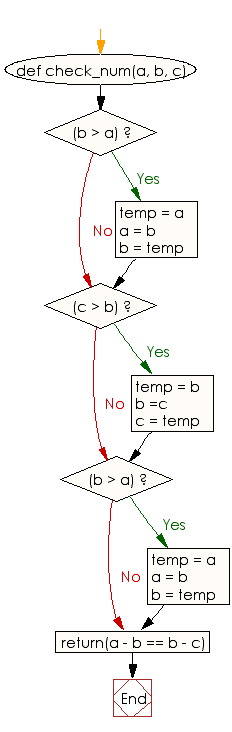﻿ Ruby Basic exercises: Check three given integers and return true if the three values are evenly spaced - w3resource# Ruby Basic Exercises: Check three given integers and return true if the three values are evenly spaced

## Ruby Basic: Exercise-55 with Solution

Write a Ruby program to check three given integers (one of them is small, one is medium and one is large) and return true if the three values are evenly spaced, so the difference between small and medium is the same as the difference between medium and large.

Ruby Code:

``````def check_num(a, b, c)
if(b > a)
temp = a
a = b
b = temp
end

if(c > b)
temp = b
b =c
c = temp
end

if(b > a)
temp = a
a = b
b = temp
end
return(a - b == b - c)
end
print check_num(5, 10, 15),"\n"
print check_num(2, 3, 11),"\n"
``````

Output:

```true
false
```

Flowchart:Ruby Code Editor: